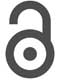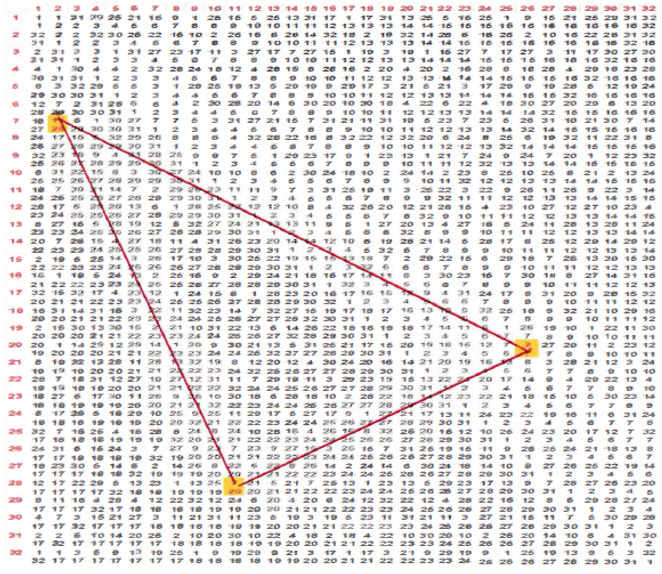## Volume 14 - Issue 4

Mini ReviewCreative Commons, CC-BY

# The Fingerprints of President Richard Milhous Nixon

*Corresponding author: Andrés J Washington, Department of Forensic Medicine and Deontology, Faculty of Medicine, Medical University, NY, USA.

Received: October 14, 2021; Published: October 22, 2021

#### Abstract

The name Richard Nixon, 1913-1994 will spark an interest of discussion when mentioned. There is however, another chapter to be explored, the president’s fingerprints. Forensic Science tells us that all fingerprints are attributed to a single individual. However, discovery of the frequency of fingerprint classifications can reveal additional information about ability, talent or mental elevation in that there is a correlation to this aspect of humanity. Mental elevation is the focus of my interest.  And we cannot deny that the former president was brilliant in many ways. President Nixon’s number 2, 7 and 9 digits displayed fingerprint classifications unique enough to be worth mentioning.

Keywords: Richard M Nixon, Fingerprints, Radial Loop, Central Pocket Loop, NCIC FPC, Frequencies

#### President Nixon’s outstanding frequencies are as follows:

Digit # 7 is a Central Pocket Loop Type Whorl pattern with an outer tracing. The NCIC FPC is CO .
Note that it has a radial profile!
The frequency of CO on the # 7 digit is the following:
367016/ 17951192 = 2.04452161171247/100
2.04452161171247

In words = two and four trillion four hundred fifty- two billion one hundred sixty-one million one hundred seventy-one thousand two hundred forty-seven hundred-trillionths  (Figure 1).
Digit # 9 is a Central Pocket Loop Type Whorl pattern with an inner tracing. The NCIC FPC is CI.
The frequency of CI on the # 9 digit is the following:
1482915/17951192 = 8.2608163290772/100
8.2608163290772

In words = eight and two trillion six hundred eight billion one hundred sixty-three million two hundred ninety thousand seven hundred seventy-two ten-trillionths (Figure 2).
In addition, the number two digit is a Radial loop with a ridge count of 17. The NCIC FPC is 67.
The frequency is the following:
176202/17951192 = 0.9815615586976063/100
0.9815615586976063
In words = nine quadrillion eight hundred fifteen trillion six hundred fifteen billion five hundred eighty-six million nine hundred seventy-six thousand sixty-three ten-quadrillionths (Figures 3&4).

The above fingerprint record provides a primary classification of 9 over 4 because different numerical values were assigned to the ten digits. In the above calculation arrangement, the value of even numbered digits over odd, instead of right hand over left are assigned. This was so that a greater distribution of primary classifications could be accomplished for filing. The count of 1 is added to the value of the numerator and denominator for consistency (Figure 5).

In the Fingerprint Geometric Analysis model, the use of the Primary Classification from the Henry System of Fingerprint Classification and Filing shows the total numerical values assigned to the ten digits, right hand as numerator over left hand as denominator instead of the total value of even numbered digits as the numerator over the total value of odd numbered digits as the denominator. The objective here is not filing but to create a geometric design to represent primary classifications .

When taking both male and female frequencies into account digits with a high frequency of whorls are assigned a lower numerical value. Digits with a low frequency of whorls are assigned a higher numerical value. This is to make all primary classifications more compatible with digit whorl frequencies.

Primary Classification First Reference Sequence (Chart 2)
President Nixon’s Primary Classification is 2 over 7, it is displayed as 2/7 on the Primary Classification First Reference Sequence.
Included classifications represent individuals compatible in working together. Intersected classifications are not compatible with those in which the classifications are included. Encompassed classifications can be controlled by those of the primary classifications of 2/7, 11/28 and 7/20. The geometric representation for 2/7 is displayed on the Primary Classification First Reference Sequence (Figure 6) (Chart 3).
An added mention on the central pocket frequencies:
Here we draw our attention to the two Central Pocket Loop Type Whorl patterns on digits # 7 and #9. They are opposite to one another in that digit # 7 has an outer tracing and is radial in formation while digit # 9 has an inner tracing and is ulnar in formation (Figure 7).Chart 2:
Note*:
Includes: Primary Classifications 2/7, 11/28 and 7/20.
Encompasses: All classifications within the red line border.
Intersects: All classifications crossed by a red line.

#### Conclusion

It should be noted that the frequency of whorl patterns for the male population shows a higher frequency to lower frequency of whorls to be the same for both right and left hand. Thus, the numerical values assigned to each digit is the same for both the right and left hand. The values are: 1=2, 2=4, 3=8, 4=1, 5=16, 6=2, 7=4, 8=8, 9=1 and digit # 10=16.
Additionally, numerical values for the female digits are assigned according to an equal standard. Thus, the numerical values assigned to the female ten digits are as follows: 1=1, 2=4, 3=8, 4=2, 5=16, 6=1, 7=4, 8=8, 9=2 and digit # 10=16.
However, when using Primary Classification Analysis in the Fingerprint Geometric Analysis model, the frequencies of both male and female whorl patterns are combined to discover a total distribution of whorl type patterns. Thus, the numerical values for male and female are as follows: 1=2, 2=4, 3=8, 4=1, 5=16, 6=1, 7=4, 8=8, 9=2 and digit # 10=16.
It must be pointed out that in the aforementioned description of male and female frequencies, the male frequency values are coincidental to the right hand and the female frequency values are coincidental to the left hand! (Figures 8&9).